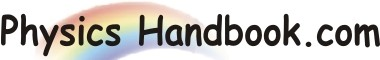HOME TOPICS DEFINITIONS TABLES LAWS INVENTIONS EXPERIMENTS QUIZ VIDEOS
 Coulomb's Law The electrostatic force between two point charges is directly proportional to the product of their magnitudes and inversely proportional to the square of the distance between their centers. This force will be repulsive or attractive depending upon whether the charges are alike or unlike charges. The force always acts along line joining the centers of the two charges. Consider two point charges q1 and q2 held r distance apart in vacuum. According to Coulomb's law, the magnitude of electrostatic force between the charges is given by: F ∞ q1 x q2 / r2 or   F  =  k x ( q1 x q2 / r2 ) Where k is a constant whose magnitude depend upon the system of units employed and the nature of medium in which charges are placed in vacuum, k  =  1 / ( 4π x ε0 )     =  1 / ( 4π x 8.854 x 10-12 )     =  9 x 109 where ε0  =  Absolute permittivity of free space  =  8.854 x 10-12 C2 N-1 m-2 F  =  ( 1 / 4π x ε0 ) x ( q1 x q2 / r2 ) or   F  =  9 x 109 x ( q1 x q2 / r2 )